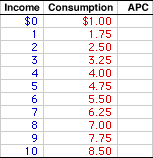Sunday  June 26, 2022
 AmosWEB means Economics with a Touch of Whimsy!REGRESSIVE TAX: A tax in which people with more income pay a smaller percentage in taxes. A regressive tax is given by this example--You earn \$10,000 a year and your boss gets \$20,000. You pay \$2,000 in taxes (20 percent) while your boss also pays \$2,000 in taxes (10 percent). Examples of regressive taxes abound (is this surprising given the political clout of the wealthy?), including sales tax, excise tax, and Social Security tax.AVERAGE PROPENSITY TO CONSUME:

The proportion of household income that is used for consumption expenditures. The average propensity to consume (abbreviated APC) is really nothing more than average consumption. Together with the average propensity to save, it indicates how a given level of income is divided between consumption and saving. A related consumption measure is the marginal propensity to consume.
The average propensity to consume (APC) indicates what the household sector does with income. The APC indicates the portion of income that is used for consumption expenditures. If, for example, the APC is 0.9, then 90 percent of income goes for consumption.

The standard formula for calculating average propensity to consume (APC) is:

 APC = consumptionincome
Consumption ScheduleA consumption schedule, such as the one presented to the right, provides data that can be used to run through a few APC calculations. The first column in this schedule presents household income, ranging from \$0 to \$10 trillion. The second column presents consumption expenditures, ranging from \$1 to \$8.5 trillion. The task at hand is to derive the average propensity to consume at each income level.

The average propensity to consume is calculated by dividing consumption in the second column by income in the first column. Beginning near the top of the schedule, if household income is \$1 trillion, then consumption is \$1.75 trillion, giving an average consumption of 1.75.

Running the numbers through the APC formula gives:

 APC = consumptionincome = \$1.75\$1 = 1.75
Similar calculations can be performed for each income level. For example, if income from \$4, then consumption is also \$4 trillion and the APC is equal 1 (\$4/\$4). If income is \$8 trillion, the consumption is \$7 trillion and the APC is equal to 0.88 (\$7/\$8). To display all average propensity to consume values, click the [APC] button.

The prime conclusion from a quick look at the numbers is that APC declines as income increases. The APC is 1.75 for \$1 trillion of income, then declines to 0.85 for \$10 trillion of income. In other words, APC is not constant.

The APC declines due to autonomous consumption and induced consumption. Autonomous consumption is the \$1 trillion of consumption that takes place if income is zero. Induced consumption is the increase in consumption that occurs due to an increase in income.

Because consumption is positive when income is zero, consumption is necessarily greater than income at low income levels, meaning the APC is greater than one. Moreover, while consumption is induced as income increases, the fundamental psychological law means that consumption increases less than income. As such, because the increment in consumption is less than the increment in income, the average declines. This is an application of the average-marginal relation.

The average propensity to consume is one of four related measures. The other three are average propensity to save, marginal propensity to consume, and marginal propensity to save.

• Average Propensity to Save: This is the proportion of household income that is used for saving. Abbreviated APS, this is really nothing more than average saving. Together with the average propensity to consumption, it indicates how a given level of income is divided between consumption and saving.

• Marginal Propensity to Consume: This is the change in consumption resulting from a change in income. Abbreviated MPC, this indicates the proportion of additional household income that is used for consumption. It quantifies the fundamental psychological law and is the most important of these four measures. The MPC is the slope of the consumption line, key to the slope of the aggregate expenditures line, and affects the magnitude of the multiplier.

• Marginal Propensity to Save: This is the change in saving resulting from a change in income. Abbreviated MPS, this indicates the proportion of additional household income that is used for saving. It is the flip side of the marginal propensity to consume, and thus also quantifies the fundamental psychological law. The MPS is the slope of the saving line, which enters into the injections-leakages model. The multiplier is also related to the MPS.

 <= AVERAGE PRODUCT CURVE AVERAGE PROPENSITY TO SAVE =>Recommended Citation:

AVERAGE PROPENSITY TO CONSUME, AmosWEB Encyclonomic WEB*pedia, http://www.AmosWEB.com, AmosWEB LLC, 2000-2022. [Accessed: June 26, 2022].

Check Out These Related Terms...

Or For A Little Background...

And For Further Study...
Search Again?PINK FADFLY[What's This?] Today, you are likely to spend a great deal of time calling an endless list of 800 numbers trying to buy either a package of blank rewritable CDs or yellow cotton balls. Be on the lookout for cardboard boxes.Your Complete ScopeThe standard "debt" notation I.O.U. does not mean "I owe you," but actually stands for "I owe unto...""You can't use up creativity. The more you use, the more you have. "-- Maya Angelou, poetICCInternational Chamber of CommerceA PEDestrian's Guide Xtra CreditTell us what you think about AmosWEB. Like what you see? Have suggestions for improvements? Let us know. Click the User Feedback link.| | | | | | | | | | |
| | | |

Thanks for visiting AmosWEB